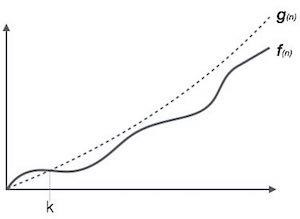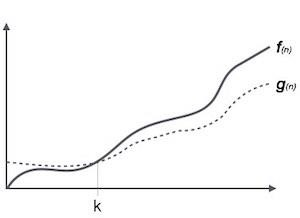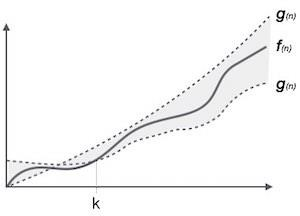# Python - Algorithm Types

The efficiency and accuracy of algorithms have to be analysed to compare them and choose a specific algorithm for certain scenarios. The process of making this analysis is called Asymptotic analysis. It refers to computing the running time of any operation in mathematical units of computation.

For example, the running time of one operation is computed as f(n) and may be for another operation it is computed as g(n2). This means the first operation running time will increase linearly with the increase in n and the running time of the second operation will increase exponentially when n increases. Similarly, the running time of both operations will be nearly the same if n is significantly small.

Usually, the time required by an algorithm falls under three types −

• Best Case − Minimum time required for program execution.

• Average Case − Average time required for program execution.

• Worst Case − Maximum time required for program execution.

## Asymptotic Notations

The commonly used asymptotic notations to calculate the running time complexity of an algorithm.

• Ο Notation

• Ω Notation

• θ Notation

### Big Oh Notation, Ο

The notation Ο(n) is the formal way to express the upper bound of an algorithm's running time. It measures the worst case time complexity or the longest amount of time an algorithm can possibly take to complete.For example, for a function f(n)

Ο(f(n)) = { g(n) : there exists c > 0 and n0 such that f(n) ≤ c.g(n) for all n > n0. }


### Omega Notation, Ω

The notation Ω(n) is the formal way to express the lower bound of an algorithm's running time. It measures the best case time complexity or the best amount of time an algorithm can possibly take to complete.For example, for a function f(n)

Ω(f(n)) ≥ { g(n) : there exists c > 0 and n0 such that g(n) ≤ c.f(n) for all n > n0. }


### Theta Notation, θ

The notation θ(n) is the formal way to express both the lower bound and the upper bound of an algorithm's running time. It is represented as follows −θ(f(n)) = { g(n) if and only if g(n) =  Ο(f(n)) and g(n) = Ω(f(n)) for all n > n0. }


## Common Asymptotic Notations

A list of some common asymptotic notations is mentioned below −

 constant − Ο(1) logarithmic − Ο(log n) linear − Ο(n) n log n − Ο(n log n) quadratic − Ο(n2) cubic − Ο(n3) polynomial − nΟ(1) exponential − 2Ο(n)